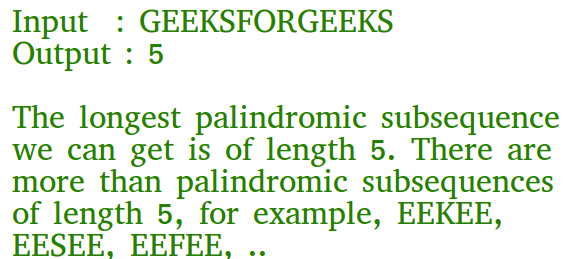PHP Program for Longest Palindromic Subsequence | DP-12

• Last Updated : 13 Aug, 2021

Given a sequence, find the length of the longest palindromic subsequence in it.As another example, if the given sequence is “BBABCBCAB”, then the output should be 7 as “BABCBAB” is the longest palindromic subsequence in it. “BBBBB” and “BBCBB” are also palindromic subsequences of the given sequence, but not the longest ones.

1) Optimal Substructure:
Let X[0..n-1] be the input sequence of length n and L(0, n-1) be the length of the longest palindromic subsequence of X[0..n-1].
If last and first characters of X are same, then L(0, n-1) = L(1, n-2) + 2.
Else L(0, n-1) = MAX (L(1, n-1), L(0, n-2)).
Following is a general recursive solution with all cases handled.

Dynamic Programming Solution

PHP

 \$y)? \$x : \$y; } // Returns the length of the// longest palindromic// subsequence in seqfunction lps(\$str){\$n = strlen(\$str);\$i; \$j; \$cl; // Create a table to store// results of subproblems\$L[][] = array(array());  // Strings of length 1 are// palindrome of length 1for (\$i = 0; \$i < \$n; \$i++)    \$L[\$i][\$i] = 1;     // Build the table. Note that    // the lower diagonal values    // of table are useless and    // not filled in the process.    // The values are filled in a    // manner similar to Matrix    // Chain Multiplication DP    // solution (See    // cl is length of substring    for (\$cl = 2; \$cl <= \$n; \$cl++)    {        for (\$i = 0; \$i < \$n - \$cl + 1; \$i++)        {            \$j = \$i + \$cl - 1;            if (\$str[\$i] == \$str[\$j] &&                            \$cl == 2)            \$L[\$i][\$j] = 2;            else if (\$str[\$i] == \$str[\$j])            \$L[\$i][\$j] = \$L[\$i + 1][\$j - 1] + 2;            else            \$L[\$i][\$j] = max(\$L[\$i][\$j - 1],                             \$L[\$i + 1][\$j]);        }    }     return \$L[\$n - 1];} // Driver Code\$seq = 'GEEKS FOR GEEKS';\$n = strlen(\$seq);echo "The length of the " .      "LPS is ", lps(\$seq); // This code is contributed// by shiv_bhakt.?>
Output
The length of the LPS is 7

Please refer complete article on Longest Palindromic Subsequence | DP-12 for more details!

My Personal Notes arrow_drop_up